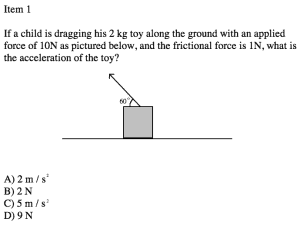# Free MCAT Practice Question – Physics

Mastering the Physical Sciences portion of the MCAT first requires mastering the fundamental science concepts that the MCAT will test.

By far, the physics questions the MCAT most likes to ask relate to kinematics and forces. Try your hand at this sample problem (click to enlarge):Explanation

Like most questions on the MCAT, this one can be approached a couple of different ways.

First, if you have no idea what you’re doing, you’re going to have to guess. The MCAT covers a huge array of topics, and sometimes we’ll see a topic we just didn’t get time to study. If that happens, don’t panic. It’s okay to guess – in fact, you have to! Don’t leave anything blank on the test! If you’re going to have to guess, guess smart.

Try to avoid picking answers that are just the simple addition or subtraction of numbers listed in the question itself. For example, in this question you probably shouldn’t guess 9, since that’s just the subtraction of the 10 and the 1 listed in the question. That’s a “panic answer” and the MCAT writers put it in there as a trap. Just guess between A, B, C and move on quickly. There’s more questions to get right!

Next, let’s say you remember a bit of physics but don’t really know how to solve this question. It’s hugely important on the test to be familiar with units. In fact, I routinely tell my tutoring students that if they learn nothing else in physics, they need to LEARN UNITS! In this question, we’re asked for acceleration. Two of the answer choices have the units listed as Newtons. That’s wrong! Acceleration is in meters per seconds squared. If you know your units, you can quickly eliminate B and D. Just pick either A or C and move on.

Finally, to answer this question down you’re going to have to break down the applied 10N force. The child is sliding the block horizontally along the floor, so you need the horizontal component of the applied force. Remember that you use cos to find the horizontal components of force. The equation would thus be:

Fx = 10N x cos 60 = 10 x 0.5 = 5 N

We’re told that the frictional force is 1 N. The frictional force would oppose the applied force, so we would find the net force by subtracting friction:

F net = 5 N – 1 N = 4 N

Now that we know the net force, we can use Newton’s Second Law, F = ma, to find the answer. This is a biggie! F=ma is probably the most important equation in all of MCAT physics.

F = ma
4 N = 2 kg x a
a = 2

MCAT is a registered trademark of the Association of American Medical Colleges (AAMC), which is not affiliated with Blueprint.